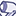# QlikView App Dev

Discussion Board for collaboration related to QlikView App Development.

Announcements
Join this live chat April 6, 10AM EST - QlikView to Qlik Sense REGISTER
cancel
Showing results for
Search instead for
Did you mean:Contributor III

## Use a field in set analysis comparison

I have this sum expression that I need to find all items that between 2 dates.  The issue is that different items have a different date that I need to look back. Here is the expression:

Sum({DateKey={"<=\$(vMax_SO_Closed_GlobalDateNum)>\$(=vMax_SO_Closed_GlobalDateNum-DaysTillDead)"}>}  [Actual Quantity])

vMax_SO_Closed_GlobalDateNum is a variable that gives the highest date in the file

DaysTillDead is a field in the Item Class Table, so all items in the Chicken class will have a certain number and all the items in the Vegetables class will have a different number

As examples of what they should be:

Sum({DateKey={"<=\$(vMax_SO_Closed_GlobalDateNum)>\$(=vMax_SO_Closed_GlobalDateNum-30)"}>}  [Actual Quantity])

or

Sum({DateKey={"<=\$(vMax_SO_Closed_GlobalDateNum)>\$(=vMax_SO_Closed_GlobalDateNum-14)"}>}  [Actual Quantity])

The problem is when I use the field, the second half of the DateKey expression doesn't work and I get this:

Sum({DateKey={"<=\$(vMax_SO_Closed_GlobalDateNum)"}>}  [Actual Quantity])

How do I get this to work? What do I need to do?  I don't think I can use a variable because it is different depending on the item.

3 RepliesMVP

Would you be able to share few rows of data with the expected output to understand your issue better? Also, it seems that you might need Aggr() function with if condition rather than using set analysis because set analysis is evaluated once per chart.Contributor III
Author

okay, I didn't know that about set analysis but here is the full expression:

if(

(isNull(ParentItem_Flag) AND Sum({

DateKey={"<=\$(vMax_SO_Closed_GlobalDateNum)>\$(=vMax_SO_Closed_GlobalDateNum-DaysTillDead)"}>}  [Actual Quantity])=0)

,

sum([Case qty on hand])

)

How would I change the if to test for between those two dates should equal 0.  That is why I was using set analysis.MVP

Sorry sir, but looking at the expression doesn't give me too much information as to what you have and what you want. As I asked earlier, would be able to provide few rows of data with the expected output to understand your issue better?

Best,

Sunny